# Review: SRCNN (Super Resolution)

In this story, a very classical super resolution technique, Super-Resolution Convolutional Neural Network (SRCNN) [1–2], is reviewed. In deep learning or convolutional neural network (CNN), we usually use CNN for image classification. In SRCNN, it is used for single image super resolution (SR) which is a classical problem in computer vision.

In brief, with better SR approach, we can get a better quality of a larger image even we only get a small image originally.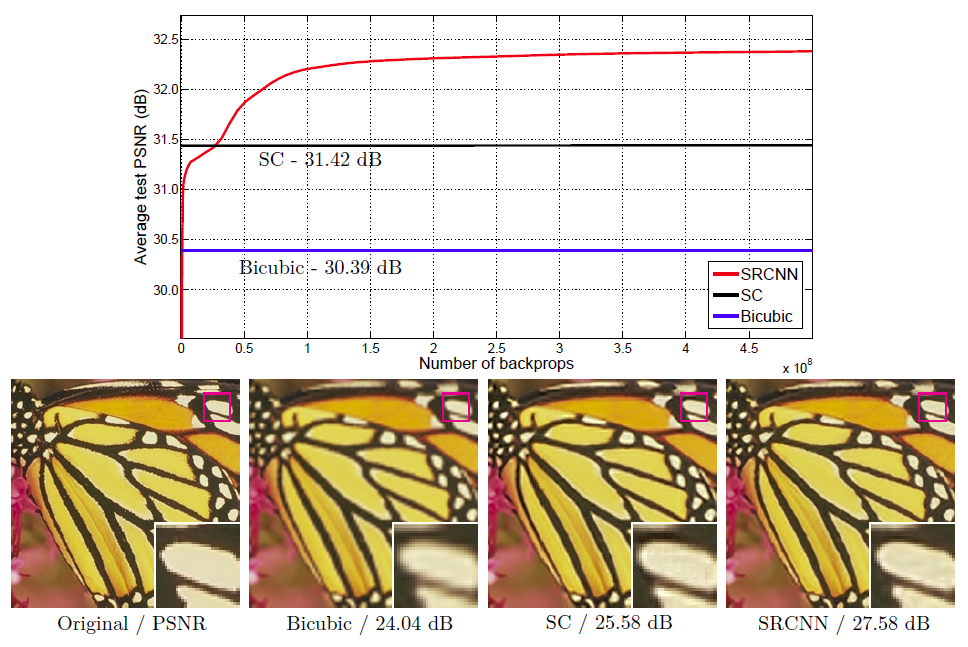SRCNN

We can see from the above figure that, with SRCNN, PSNR of 27.58 dB is obtained which is much better than the classical non-learning based Bicubic and sparse coding (SC) which was and still is also a very hot research topic.

SRCNN is published in 2014 ECCV  and 2016 TPAMI  papers with both about 1000 citations when I was writing this story. (Sik-Ho Tsang @ Medium)

### What are covered

1. The SRCNN Network
2. Loss Function
3. Relationship with Sparse Coding
4. Comparison with State-of-the-art Approaches
5. Ablation Study

### 1. The SRCNN Network

In SRCNN, actually the network is not deep. There are only 3 parts, patch extraction and representation, non-linear mapping, and reconstruction as shown in the figure below: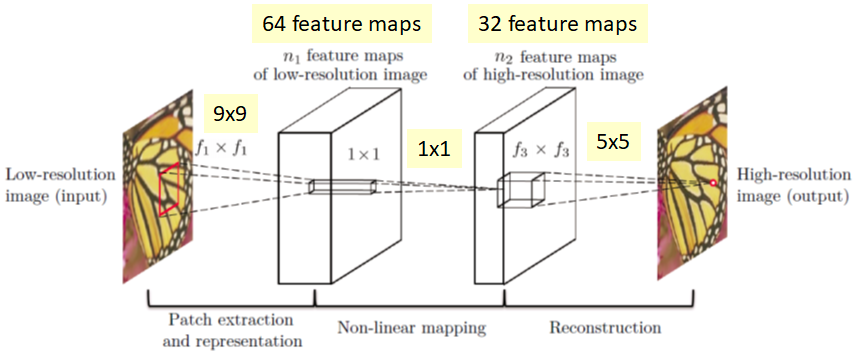SRCNN Network

#### 1.1 Patch Extraction and Representation

It is important to know that the low-resolution input is first upscale to the desired size using bicubic interpolation before inputting to SRCNN network. Thus,
X: Ground truth high-resolution image
Y: Bicubic upsampled version of low-resolution image

And the first layer perform a standard conv with Relu to get F1(Y).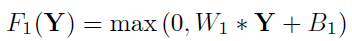The first Layer

Size of W1: c×f1×f1×n1
Size of B1: n1

where c is number of channels of the image, f1 is the filter size, and n1 is the number of filters. B1 is the n1-dimensional bias vector which is just used for increasing the degree of freedom by 1.

In this case, c=1, f1=9, n1=64.

#### 1.2 Non-Linear Mapping

After that, a non-linear mapping is performed.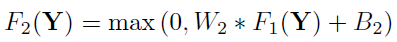The second layer

Size of W2: n1×1×1×n2
Size of B2: n2

It is a mapping of n1-dimensional vector to n2-dimensional vector. When n1>n2, we can imagine something like PCA stuffs but in a non-linear way.

In this case, n2=32.

This 1×1 actually is a 1×1 convolution suggested in Network In Network (NIN)  as well. In NIN, 1×1 convolution is suggested to introduce more non-linearlity to improve the accuracy. It is also suggested in GoogLeNet  for reducing the number of connections. (Please visit my review for 1×1 convolution in GoogLeNet if interested.)

Here, it is used for mapping low-resolution vector to high-resolution vector.

#### 1.3 Reconstruction

After mapping, we need to reconstruct the image. Hence, we do conv again.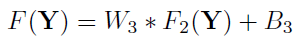The third layer

Size of W3: n2×f3 ×f3×c
Size of B3: c

### 2. Loss Function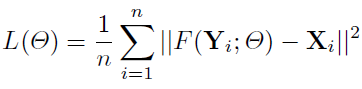Loss function

For super resolution, the loss function L is the average of mean square error (MSE) for the training samples (n), which is a kind of standard loss function.

### 3. Relationship with Sparse Coding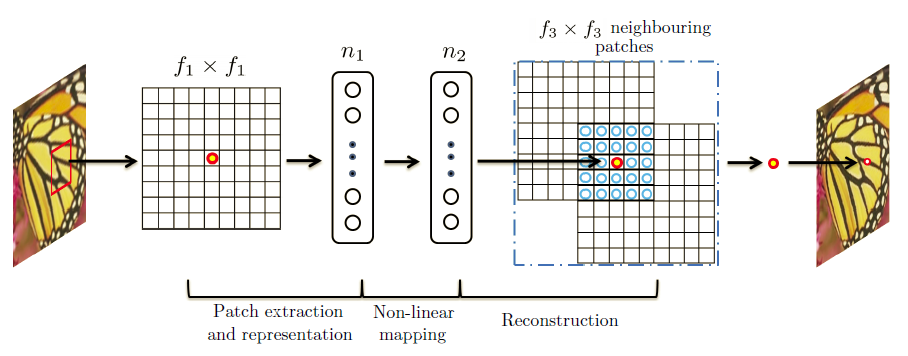Sparse Coding

For Sparse Coding (SC), in the view of convolution, the input image is conv by f1 and project to onto a n1-dimensional dictionary. n1=n2 usually is the case of SC. Then mapping of n1 to n2 is done with the same dimensionality without reduction. It is just like a mapping of low-resolution vector to high-resolution vector. Then each patch is reconstructed by f3. And overlapping patches are averaged instead of adding together with different weights by convolution.

### 4. Comparison with State-of-the-art Approaches

91 training images provide roughly 24,800 sub-images with stride 14 and Gaussian blurring. And takes 3 days for training on a GTX 770 GPU with 8×10⁸ backpropagations.

Different scales from 2 to 4 are tested.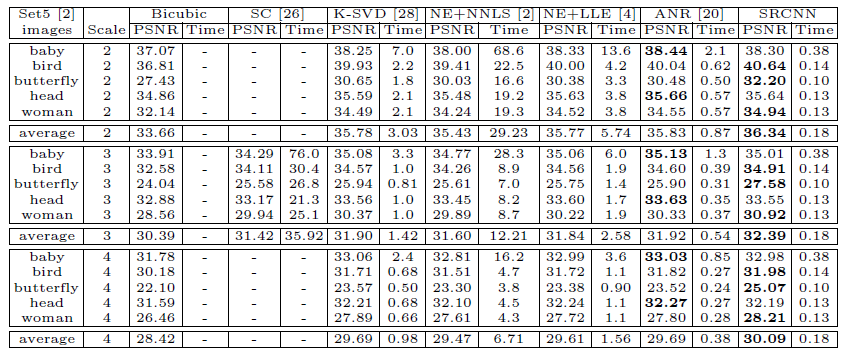PSNR for Set15 dataset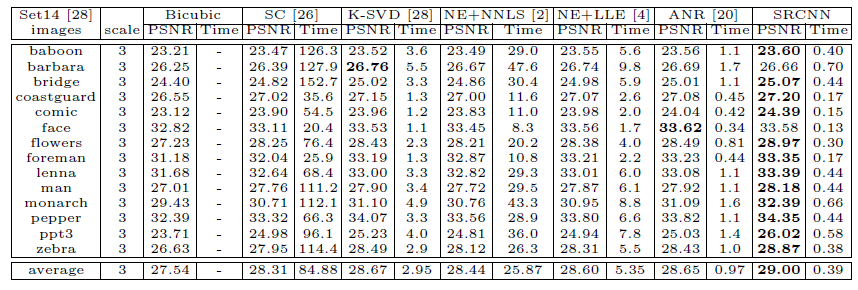PSNR for Set14 dataset

SRCNN obtains the highest average PSNR.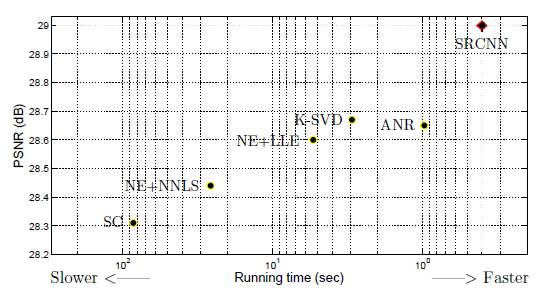PSNR against Time

The righter, the faster, the higher, the better quality.
And SRCNN is at the top right corner which has the best performance.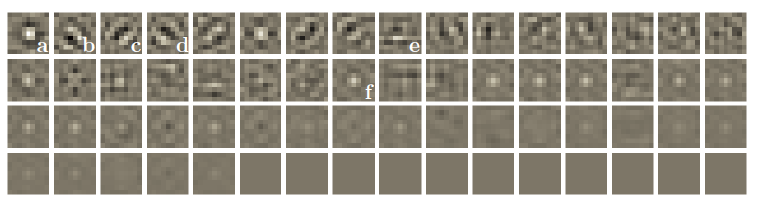Visualization of first-layer filters

Some visual qualities:

### 5. Ablation Study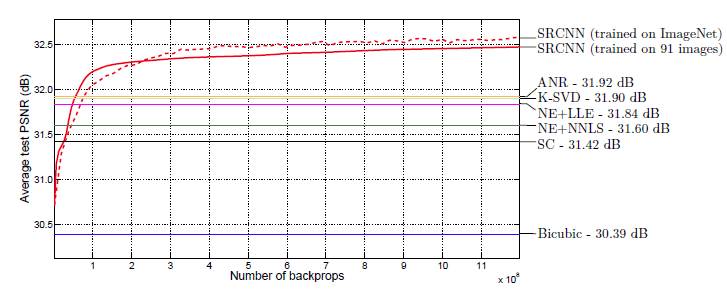Training from ImageNet vs Training from 91 images

If SRCNN trained using 395,909 images which is partially from ILSVRC 2013 ImageNet detection training dataset, the result is better than just trained from 91 images.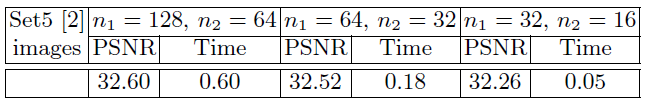Different number of n1 and n2, Trained from ImageNet and Test on Set5

The larger n1 and n2, the higher the PSNR. It is normal as more filters, it should be better.

Also, with larger filter size, it also leads to a little better results. (But actually, there are only 3 layers, it is not sufficient enough to prove this. They should increase the layers as well. If there are more layers, larger filters can be replaced by several small filters.)

SRCNN contains only 3 layers. It is a easy and worth to read paper. So, it is also a paper to act as a starting point for learning deep learning or CNN! :)